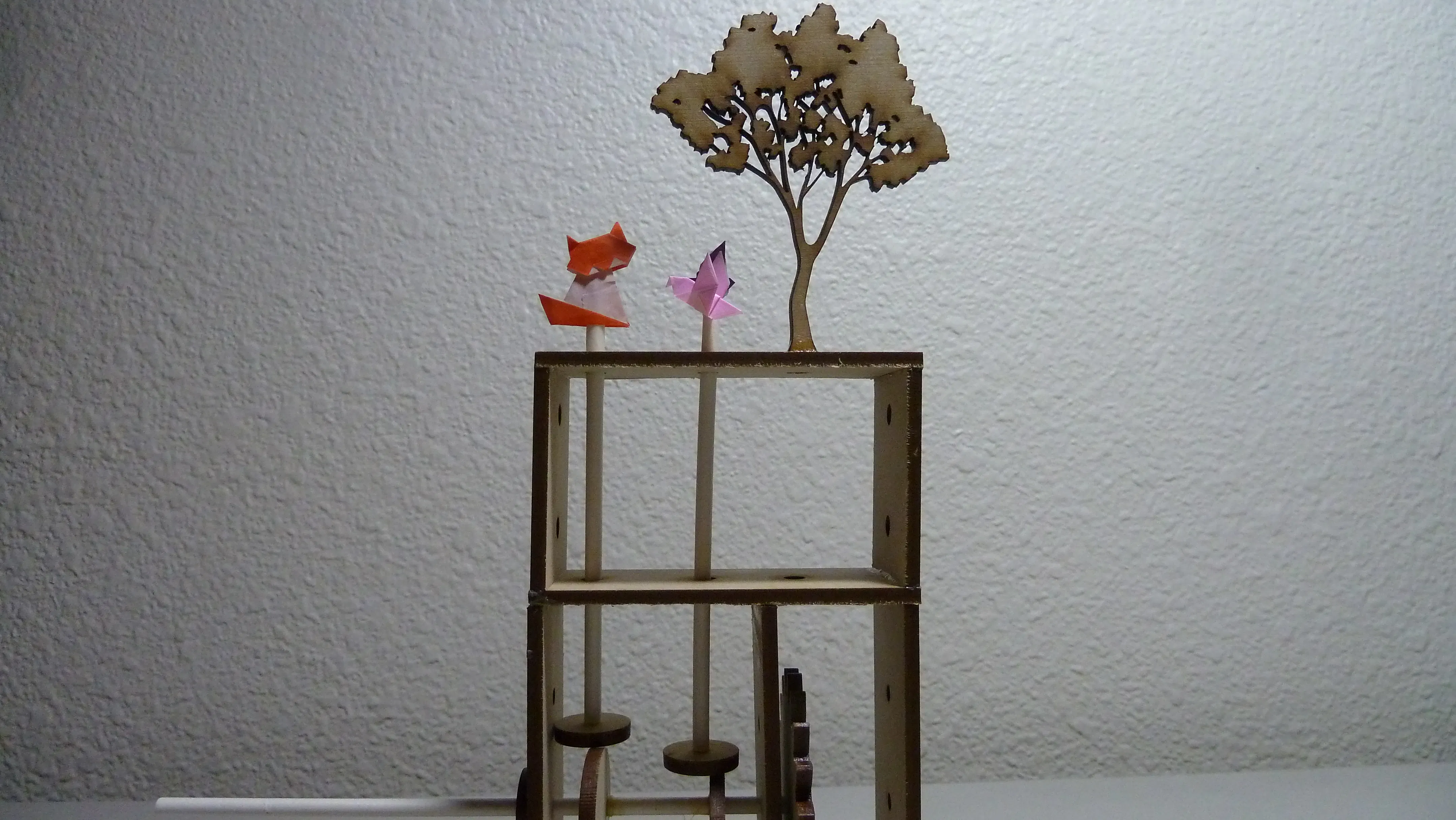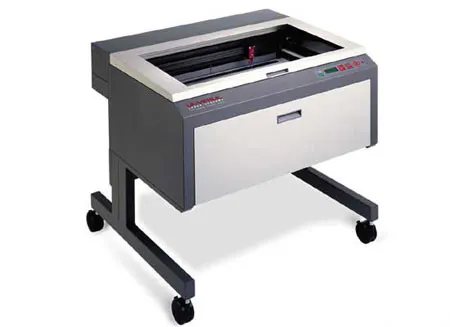Created September 28, 2015

# Cat & Birds Automata + Arduino LED Button

Laser Cut Gear Automata

BeginnerFull instructions provided648## Things used in this project

### Hardware componentsArduino UNO
×1

### Hand tools and fabrication machinesLaser cutter (generic)

## Code

### Example 1:

C/C++
Turn on an LED while the button is pressed
```// Turn on LED while the button is pressed
// Massimo Banzi, from "Getting Started with Arduino"

const int LED = 13;   // the pin for the LED
const int BUTTON = 7; // the input pin where the
// pushbutton is connected
int val = 0;          // val will be used to store the state
// of the input pin

void setup() {
pinMode(LED, OUTPUT);   // tell Arduino LED is an output
pinMode(BUTTON, INPUT); // and BUTTON is an input
}

void loop(){

// check whether the input is HIGH (button pressed)
if (val == HIGH) {
digitalWrite(LED, HIGH); // turn LED ON
} else {
digitalWrite(LED, LOW);
}
}
```

### Example 2:

C/C++
Press button to turn on LED, press again to turn off, innocent version
```// Press button to turn on LED, press again to turn off, innocent version
// Massimo Banzi, from "Getting Started with Arduino"
const int LED = 13; // the pin for the LED
const int BUTTON = 7; // the input pin where the
// pushbutton is connected
int val = 0;                    // val will be used to store the state
// of the input pin
int state = 0;                // 0 = LED off while 1 = LED on

void setup() {
pinMode(LED, OUTPUT);     // tell Arduino LED is an output
pinMode(BUTTON, INPUT); // and BUTTON is an input
}

void loop() {

// check if the input is HIGH (button pressed)
// and change the state
if (val == HIGH) {
state = 1 - state;
}

if (state == 1) {
digitalWrite(LED, HIGH); // turn LED ON
} else {
digitalWrite(LED, LOW);
}
}
```

### Example 3:

C/C++
Only change LED state on button transition
```// Only change LED state on button transition
// Massimo Banzi, from "Getting Started with Arduino"
const int LED = 13;   // the pin for the LED
const int BUTTON = 7; // the input pin where the
// pushbutton is connected
int val = 0;     // val will be used to store the state
// of the input pin
int old_val = 0; // this variable stores the previous
// value of "val"
int state = 0;   // 0 = LED off and 1 = LED on

void setup() {
pinMode(LED, OUTPUT);   // tell Arduino LED is an output
pinMode(BUTTON, INPUT); // and BUTTON is an input
}
void loop(){
// yum, fresh

// check if there was a transition
if ((val == HIGH) && (old_val == LOW)){
state = 1 - state;
}

old_val = val;  // val is now old, let's store it

if (state == 1) {
digitalWrite(LED, HIGH); // turn LED ON
} else {
digitalWrite(LED, LOW);
}
}
```

## Credits

### Jennifer Chen

13 projects • 11 followers